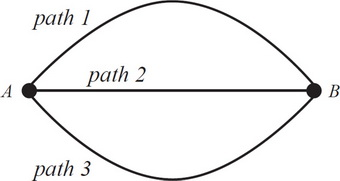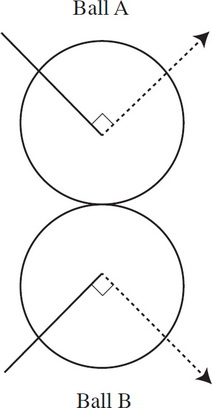# AP Physics 1 Practice Test 8

### Test Information10 questions18 minutes

1. A wooden block experiences a frictional force, f, as it slides across a table. If a block of the same material with half the height and twice the length were to slide across the table, what would be the frictional force it experienced?

2. Two objects, a sphere and a block of the same mass, are released from rest at the top of an inclined plane. The sphere rolls down the inclined plane without slipping. The block slides down the plane without friction. Which object reaches the bottom of the ramp first?

3.In the diagram above, a mass m starting at point A is projected with the same initial horizontal velocity v0 along each of the three tracks shown here (with negligible friction) sufficient in each case to allow the mass to reach the end of the track at point B. (Path 1 is directed up, path 2 is directed horizontal, and path 3 is directed down.) The masses remain in contact with the tracks throughout their motions. The displacement A to B is the same in each case, and the total path length of path 1 and 3 are equal. If t1,t2, and t3 are the total travel times between A and B for paths 1, 2, and 3, respectively, what is the relation among these times?

4. An object of mass m1 experiences a linear, elastic collision with a stationary object of unknown mass. In addition to m1, what is the minimum necessary information that would allow you to determine the mass of the second object?

5. A block is dragged along a table and experiences a frictional force, f, that opposes its movement. The force exerted on the block by the table is

6. A box of mass m is sitting on an incline of 45° and it requires an applied force f up the incline to get the box to begin to move. What is the maximum coefficient of static friction?

7.The graph above shows the velocities of two objects undergoing a head-on collision. Given that Object 1 has 4 times the mass of Object 2, which type of collision is it?

8.The picture above depicts the collision of two balls of equal mass. Which arrow best indicates the direction of the impulse on ball A from ball B during the collision?

9. Which of the following best describes the relationship between the magnitude of the tension force, FT, in the string of a pendulum and the radial component of gravity that pulls antiparallel to the tension, Fg, radial? Assume that the pendulum is only displaced by a small amount.

10. Pretend someone actually managed to dig a hole straight through the center of the Earth all the way to the other side. If an object were dropped down that hole, which of the following would best describe its motion? Assume ideal conditions and that the object cannot be destroyed.